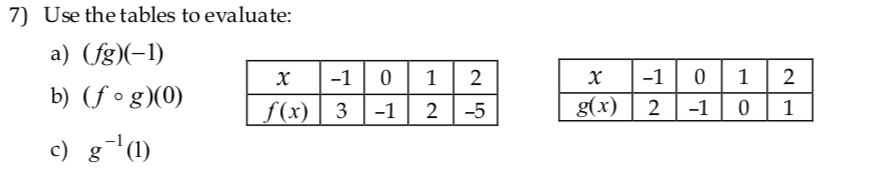# Use the tables to evaluate:a) (Ug)(-1)b) (f o g)00)e) g-0)7)x-1 0 1 2g(x) 2 10 1x-1 0 1 2

Questionhelp_outlineImage TranscriptioncloseUse the tables to evaluate: a) (Ug)(-1) b) (f o g)00) e) g-0) 7) x-1 0 1 2 g(x) 2 10 1 x-1 0 1 2 fullscreen
check_circleExpert Solution
Step 1

Calculation:

a)

Compute the value of (fg)(-1) as follows.

(fg)(-1) = f(-1) × g(-1)                               ... (1)

From the given table, the value of f(-1) and g(-1) are observed to be 3 and 2, respectively.

Now substitute f(-1) = 3 and g(-1) = 2 in equation (1).

(fg)(-1) = 3 × 2

(fg)(-1) = 6.

Step 2

b)

Compute the value of (f ⸰ g) (0) as follows.

Step 3

c)

Compute the value of g-1(1) as follows.

Assume that g-1(1) = x. Then g(x) =1.

From the given table the value of g(x)...

### Want to see the full answer?

See Solution

#### Want to see this answer and more?

Solutions are written by subject experts who are available 24/7. Questions are typically answered within 1 hour*

See Solution
*Response times may vary by subject and question
Tagged in

### Other